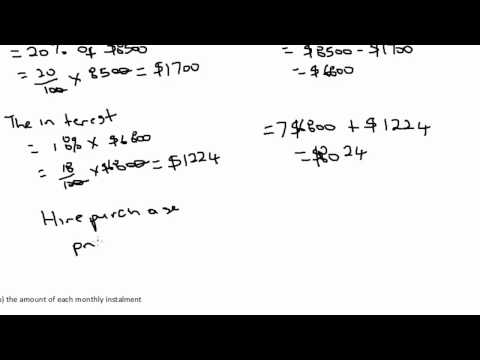## Mathematical IQ Test

Subject: General Knowledge and IQ

#### Overview

Mathematical intelligence is a strong indicator of general intelligence because many every day mental tasks require arithmetical operations even though numbers may not be involved. So, Here are some problems which you will try to solve as fast as you can...
##### Mathematical IQ Test

Mathematical Intelligence

We all require some numerical skills in our lives, whether it is to calculate our weekly shopping bill or to budget how to use our monthly income. Flexibility of thought and lateral thinking processes are a few skills which are needed in order to solve these problems. Mathematical intelligence generally represents your ability to reason and to calculate basic arithmetic computations. It also helps you to understand geometric shapes and manipulate equations. Mathematical intelligence is a strong indicator of general intelligence because many every day mental tasks require arithmetical operations even though numbers may not be involved.

1. Which number should come next in this series?

25,24,22,19,15

A. 4B. 5C. 10D. 14

Correct answer: CExplanation: The pattern decreases progressively: -1, -2, -3, -4, -52. Which number should come next in this series?

3,5,8,13,21,

A. 4B. 21C. 31D. 34

Correct answer: DExplanation: 3+5=8, 5+8=13 and so on.

3. Which number should replace the question mark?

 17 8 5 5 13 7 5 4 6 12 6 3 10 6 4 ?

A. 4B. 5C. 6D. 7

(For each row the sum of the first two columns is equal to the multiple of the last two columns)

4. Which number should replace the question mark?

 8 5 21 35 32 12 32 28 31 4 ? 28

A. 3B. -2C. -6D. 48

(For each row subtract the second column from the first column. The result is equal to the sum of the digits in the last column.)

##### Things to remember
• It includes every relationship which established among the people.
• There can be more than one community in a society. Community smaller than society.
• It is a network of social relationships which cannot see or touched.
• common interests and common objectives are not necessary for society.
##### Videos for Mathematical IQ Test##### Consumer Arithmetic Tutorial Part 1